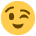# stuck on FP practice exam 2

Evening all!

I can only get £60 by doing £50 * 120%• Hi,
I can't remember the exact calculation but I'd do this by dividing 100 by the percentage margin needed, ie 20, so 5 of coursethen thinking that the 50 is only 4 of the 5 needed so I divide the 50 by 4 and add that amount on. That's written so badly but basically margin is a percentage of the total sales price (£62.50 * 20% = the 12.50 which was the amount added to the cost of £50) whereas mark-up is 20% of the cost price as you have done. Clear as mud? Hope it helps a bit!
Lucy

Ah, try the calculation for working out tax paid and gross on savings! So 50*100/80 If it was a 35% margin it would be 50*100/65 yeah?! Good luck x
• Lucy_M wrote: »
Hi,
I can't remember the exact calculation but I'd do this by dividing 100 by the percentage margin needed, ie 20, so 5 of coursethen thinking that the 50 is only 4 of the 5 needed so I divide the 50 by 4 and add that amount on. That's written so badly but basically margin is a percentage of the total sales price (£62.50 * 20% = the 12.50 which was the amount added to the cost of £50) whereas mark-up is 20% of the cost price as you have done. Clear as mud? Hope it helps a bit!
Lucy

Ah, try the calculation for working out tax paid and gross on savings! So 50*100/80 If it was a 35% margin it would be 50*100/65 yeah?! Good luck x

Hi Lucy and welcome :001_smile:

Thanks for that much appreciated.

Do you have any ideas on the difference between "profit margin" and "mark up"?thanks again
• Your welcome, yes, no problem, mark-up is basically adding the percentage of profit on to the cost price but margin is the percentage of the total price that is profit. So with your first example, for mark-up of 20% add 20% but for a margin of 20% the total price divided by 20% gives £50 cost. So with the 2nd example, for the margin, the sales price would be £80 x 100/70 =£114 (rounded). If you take 30% off £114 it gives £80 (roughly). Mark-up of 30% on cost would only be £104, £80 x 30%. Does that help? Hope so! x
• Lucy_M wrote: »
Your welcome, yes, no problem, mark-up is basically adding the percentage of profit on to the cost price but margin is the percentage of the total price that is profit. So with your first example, for mark-up of 20% add 20% but for a margin of 20% the total price divided by 20% gives £50 cost. So with the 2nd example, for the margin, the sales price would be £80 x 100/70 =£114 (rounded). If you take 30% off £114 it gives £80 (roughly). Mark-up of 30% on cost would only be £104, £80 x 30%. Does that help? Hope so! x

Thanks, this helped a lot :thumbup1: